# 1. HMM背景

## 0x2：概率图模型（probabilistic graphical model）

1. 使用有向无环图表示变量间依赖关系：有向图模型或贝叶斯网（Bayesian network）；
2. 使用无向图表示变量间的相关关秀：无向图模型或马尔科夫网（Markov network）；

## 0x5：笔者对隐马尔科夫中“隐序列”的思考

HMM的核心思想认为，任何在现实世界观测到的现象，其背后一定有一个“本质规律”在支撑这一事实，“本质规律”衍生出被我们观测到的现象。

《机器学习》周志华

# 2. 从一个虚构的例子引入马尔科夫模型讨论

## 0x1: 实验例子说明

1. 第一个骰子是我们平常见的骰子（称这个骰子为D6），6个面，每个面（1，2，3，4，5，6）出现的概率是1/6
2. 第二个骰子是个四面体（称这个骰子为D4），每个面（1，2，3，4）出现的概率是1/4
3. 第三个骰子有八个面（称这个骰子为D8），每个面（1，2，3，4，5，6，7，8）出现的概率是1/8### 1.实验假设前提

1. 每次从三个骰子里挑一个，挑到每一个骰子的概率未知；
2. 每次选的骰子对下一次选择是否有影响也是未知；
3. 对每个骰子来说抛到某一个面的概率是相等的（即骰子是没有动过手脚的）

### 3. 实验目标

1. 我们得到了这一串数字，每次抛骰子具体是3个中的哪个骰子是未知的，现在需要估计出整个10次实验中最可能的骰子类型是什么（即每次实验对应的骰子）；
2. 我们得到了这一串数字，每次抛骰子具体是3个中的哪个骰子是未知的，现在需要估计出现这个观测结果（这一串数字）的概率有多大

## 0x2: 从独立同分布假设开始 - 极简化的HMM假设

### 1. 独立同分布假设

1. 每次实验选择什么骰子是完全独立随机的（即 1/3）；
2. 每次实验和上一次实验也不存在关系，即实验之间是独立同分布的；

### 2. 我们得到了这一串数字，每次抛骰子具体是3个中的哪个骰子是未知的，现在需要估计出整个10次实验中最可能的骰子类型是什么（即每次实验对应的骰子）

P（S骰子= S1，Res结果=1 | Model） = 1 / 6

P（S骰子= S2，Res结果=1 | Model） = 1 / 4

P（S骰子= S3，Res结果=1 | Model） = 1 / 8

Res：1，S = S2
Res：6，S = S1
Res：3，S = S2
Res：5，S = S1
Res：2，S = S2
Res：7，S = S3
Res：3，S = S2
Res：5，S = S1
Res：2，S = S2
Res：4，S = S2

### 3. 我们得到了这一串数字，每次抛骰子具体是3个中的哪个骰子是未知的，现在需要估计出现这个观测结果（这一串数字序列）的概率有多大

P（Res结果=1 | Model） = P（S骰子= S1，Res结果=1 | Model） + P（S骰子= S2，Res结果=1 | Model）+ P（S骰子= S3，Res结果=1 | Model） = 1 / 6 + 1 / 4 + 1 / 8 = 13 / 24
P（Res结果=6 | Model） = P（S骰子= S1，Res结果=1 | Model） + P（S骰子= S2，Res结果=6 | Model）+ P（S骰子= S3，Res结果=6 | Model） = 1 / 6 + 0 + 1 / 8 = 7 / 24
P（Res结果=3 | Model） = P（S骰子= S1，Res结果=1 | Model） + P（S骰子= S2，Res结果=3 | Model）+ P（S骰子= S3，Res结果=3 | Model） = 1 / 6 + 1 / 4 + 1 / 8 = 13 / 24
P（Res结果=5 | Model） = P（S骰子= S1，Res结果=1 | Model） + P（S骰子= S2，Res结果=5 | Model）+ P（S骰子= S3，Res结果=5 | Model） = 1 / 6 + 0 + 1 / 8 = 7 / 24
P（Res结果=2 | Model） = P（S骰子= S1，Res结果=1 | Model） + P（S骰子= S2，Res结果=2 | Model）+ P（S骰子= S3，Res结果=2 | Model） = 1 / 6 + 1 / 4 + 1 / 8 = 13 / 24
P（Res结果=7 | Model） = P（S骰子= S1，Res结果=1 | Model） + P（S骰子= S2，Res结果=7 | Model）+ P（S骰子= S3，Res结果=7 | Model） = 0 + 0 + 1 / 8 = 1 / 8
P（Res结果=3 | Model） = P（S骰子= S1，Res结果=1 | Model） + P（S骰子= S2，Res结果=3 | Model）+ P（S骰子= S3，Res结果=3 | Model） = 1 / 6 + 1 / 4 + 1 / 8 = 13 / 24
P（Res结果=5 | Model） = P（S骰子= S1，Res结果=1 | Model） + P（S骰子= S2，Res结果=5 | Model）+ P（S骰子= S3，Res结果=5 | Model） = 1 / 6 + 0 + 1 / 8 = 7 / 24
P（Res结果=2 | Model） = P（S骰子= S1，Res结果=1 | Model） + P（S骰子= S2，Res结果=2 | Model）+ P（S骰子= S3，Res结果=2 | Model） = 1 / 6 + 1 / 4 + 1 / 8 = 13 / 24
P（Res结果=4 | Model） = P（S骰子= S1，Res结果=1 | Model） + P（S骰子= S2，Res结果=4 | Model）+ P（S骰子= S3，Res结果=4 | Model） = 1 / 6 + 1 / 4 + 1 / 8 = 13 / 24

(13 / 24) * (7/24) *  (13 / 24) * (7/24) * (13 / 24) * (1/8) * (13 / 24) * (7/24) * (13 / 24) * (13 / 24) = 7.83363029759e-05

## 0x3: 马尔科夫假设

### 1. N阶序列依赖假设

1. 从一次实验到下一次选择什么骰子是完全随机的（即 1/3 ），也即状态转移概率为 1/3；
2. 每次实验之前不再是独立同分布的，每次实验都与之前的N次实验有依赖关联；

### 5. 输出概率矩阵

六面骰（D6）产生1的输出概率是1/6。产生2，3，4，5，6的概率也都是1/6；

### 6. 我们得到了这一串数字（可见状态链已知），现在需要估计出整个10次实验中最可能的骰子类型是什么（即求隐状态序列）（复用了第一步的结果）1. 首先，不管序列多长，要从序列长度为1算起，算序列长度为1时取到每个骰子的最大概率；
2. 然后，逐渐增加长度，每增加一次长度，重新算一遍N步长度前到这个长度下最后一个位置取到每个骰子的最大概率（如果是1阶HMM只要算上一步即可），注意，不是说直接把上一步结果拿过来直接用就可以了，在转移概率不是等比随机的情况下，上一步不是概率最大的路径可能在这一步变为最大。但是好消息是，N步长度前到这个长度长度下的取到每个骰子的最大概率都算过了，计算过程中只要保存下来重新算并不难。按照这个方法，当我们算到最后一位时，就知道最后一位是哪个骰子的概率最大了。
3. 最后，我们把对应这个最大概率的序列从后往前推出来，就得到最大概率骰子序列

### 8. 我们得到了这一串数字（可见状态链已知），每次抛骰子类型已知（转换概率已知），现在需要估计出现这个观测结果的概率有多大1. D6的下一个状态是D4，D6，D8的概率都是1/3
2. D4的下一个状态是D4，D6，D8的概率都是1/3
3. D8的下一个状态是D4，D6，D8的概率都是1/3

P =

P（D6） * P（D6 -> 1） *

P（D6 -> D8）* P（D8 -> 6） *

P（D8 -> D8）* P（D8 -> 3） *

P（D8 -> D6）* P（D6 -> 5） *

P（D6 -> D4）* P（D4 -> 2） *

P（D4 -> D8）* P（D8 -> 7） *

P（D8 -> D6）* P（D6 -> 3） *

P（D6 -> D6）* P（D6 -> 5） *

P（D6 -> D4）* P（D4 -> 2） *

P（D4 -> D8）* P（D8 -> 4） =

1 / 3 * 1 / 6 * 1 / 3 * 1 / 8 * 1 / 3 * 1 / 8 * 1 / 3 * 1 / 6 * 1 / 3 * 1 / 4 * 1 / 3 * 1 / 8* 1 / 3 * 1 / 6 * 1 / 3 * 1 / 6 * 1 / 3 * 1 / 4 * 1 / 3 * 1 / 8 = 1.99389608506e-13

1. 在HMM中，隐变量是转换概率。知道了转换概率，根据实验观测结果可以最大似然推测出时序
2. HMM中，隐变量和观测变量的关系是前者决定后者的关系，即输出概率

http://blog.163.com/zhoulili1987619@126/blog/static/353082012013113191924334/
https://www.zhihu.com/question/20962240

# 3. 马尔科夫模型介绍

## 0x1：隐马尔可夫模型图结构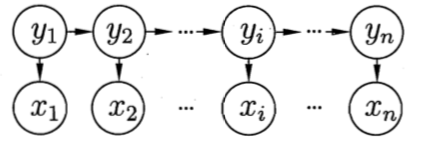## 0x2: 马尔科夫假设 - 序列依赖

### 1. 对含有序列特征的数据集建立独立同分布假设不合理### 2. 考虑当前状态和所有历史状态的依赖关系 - 一种极端的假设### 3. 一阶马尔科夫假设 - 只考虑相邻上一步的序列依赖关系#### 1）齐次马尔科夫性假设#### 2）观测独立性假设## 0x3: 隐马尔可夫模型的定义

### 1. 模型结构信息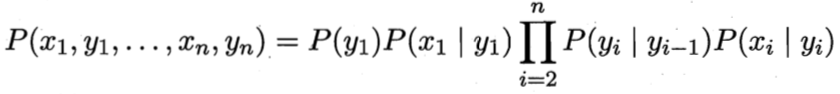### 2. 状态转移概率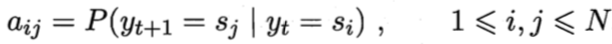表示在任意时刻 t，若状态为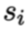，则在下一时刻状态为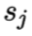的概率。

### 3. 输出观测概率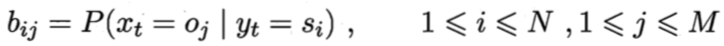表示在任意时刻 t，若状态为，则观测值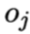被获取的概率。

### 4. 初始状态概率和A决定状态序列；B决定观测序列。

## 0x4: 隐马尔可夫模型的3个基本问题

### 3. 预测问题/解码问题 - 反推隐藏状态序列

https://www.zhihu.com/question/20962240
http://www.cnblogs.com/skyme/p/4651331.html
http://blog.csdn.net/bi_mang/article/details/52289087
https://yanyiwu.com/work/2014/04/07/hmm-segment-xiangjie.html
https://www.zhihu.com/question/20962240
https://www.zhihu.com/question/52778636
https://www.zhihu.com/question/37391215
https://www.zhihu.com/question/20551866
https://www.zhihu.com/question/28311766
https://www.zhihu.com/question/28311766
https://www.zhihu.com/question/22181185

# 4. 观测序列概率计算算法 - 马尔科夫模型中的观测序列概率计算

## 0x1: 直接计算法

O 和 I 同时出现的联合概率为：## 0x2: 前向-后向算法

### 1. 前向算法 - 一种递归算法

（1）初值：，是初始时刻的状态和观测的联合概率

（2）递推：对，有：，乘积就是时刻 t 观测到并在时刻 t 处于状态而在时刻 t + 1 到达状态的联合概率。对这个乘积在时刻 t 的所有可能的 N 个状态求累加和，其结果就是到时刻 t 观测为并在时刻 t + 1处于状态的联合概率（3）终止：### 2. 前向算法的一个具体应用举例

#### 1）计算初值

a1（1） = π1 * b1（o1）= 0.2 * 0.5 = 0.1

a1（2） = π2 * b2（o1）= 0.4 * 0.4 = 0.16

a1（3） = π3 * b3（o1）= 0.4 * 0.7 = 0.28

#### 2）递推计算 t = 2= （a1（1） * a11 + a1（2） * a21 + a1（3） * a31） * b1（o2） = （0.1 * 0.5 + 0.16 * 0.3 + 0.28 * 0.2）* 0.5 = 0.077= （a1（1） * a12 + a1（2） * a22 + a1（3） * a32） * b2（o2） = （0.1 * 0.2 + 0.16 * 0.5 + 0.28 * 0.3）* 0.6 = 0.184 * 0.6 = 0.1104= （a1（1） * a13 + a1（2） * a23 + a1（3） * a33） * b3（o2） = （0.1 * 0.3 + 0.16 * 0.2 + 0.28 * 0.5）* 0.3 = 0.202 * 0.3 = 0.0606

#### 3）递推计算 t = 3= （a2（1） * a11 + a2（2） * a21 + a2（3） * a31） * b1（o3） = （0.077 * 0.5 + 0.1104 * 0.3 + 0.0606* 0.2）* 0.5 = 0.04187= （a2（1） * a12 + a2（2） * a22 + a2（3） * a32） * b1（o3） = （0.077 * 0.2 + 0.1104 * 0.5 + 0.0606* 0.3）* 0.4 = 0.035512= （a2（1） * a13 + a2（2） * a23 + a2（3） * a33） * b1（o3） = （0.077 * 0.3 + 0.1104 * 0.2 + 0.0606* 0.5）* 0.7 = 0.052836

#### 4）终止= a3（1） + a3（2）+ a3（3） = 0.04187 + 0.035512 + 0.052836 = 0.130218

### 3. 后向算法 - 一种递推算法

（1）初始化后向概率：，对最终时刻的所有状态规定（2）后向概率的递推公式：，为了计算在时刻 t 状态为条件下时刻 t + 1之后的观测序列为的后向概率，只需考虑在时刻 t + 1所有可能的N个状态的转移概率（即项），以及在此状态下的观测的观测概率（即项），然后考虑状态之后的观测序列的后向概率（即项）（递推下去）（3）最终结果：，可以看到后向概率的最终结果表达式就是前向概率的初始化表达式《统计机器学习》李航

# 5. 隐马尔柯夫模型学习算法 - 模型参数最大似然估计

## 0x1: 监督学习方法

### 1. 转移概率矩阵的估计### 2. 观测概率矩阵的估计### 3. 初始状态概率的估计为 S 个样本中初始状态为的频率

## 0x2: 无监督学习方法 - Baum-Welch算法（EM算法）### 3. EM算法的 M 步

（1）第一项（2）第二项：（3）第三项：# 6. 隐马尔科夫隐状态估计算法 - 隐状态概率矩阵最大似然估计

## 0x2: 维特比算法

### 1. 初始化### 2. 递推：对t = 2，3，...，T### 3. 终止### 4. 最优路径回溯：对t = T - 1，T - 2，....，1## 0x3: 通过一个例子来说明维特比算法（1）初始化

（2）递推 t = 2

（3）递推 t = 3

同样，在t = 3步，还是逐步引入t = 2步的所有概率最大概率，并结合t = 3步的转移概率和观测概率（4）终止表示最优路径的概率，则，该结果是逐步递推出来的

（5）由最优路径的终点，逆向逆推最优路径序列# 7. 隐马尔科夫模型的应用

## 0x1：序列标注问题1. 开始，从4个盒子里随机选取1个盒子（1/4等概率），从这个盒子里随机抽出1个球，记录其颜色后，放回；
2. 然后，从当前盒子随机转移到下一个盒子，规则是
1）如果当前盒子是盒子1，那么下一个盒子一定是2
2）如果当前盒子是2或3，那么分别以概率0.4和0.6转移到左边或右边的盒子
3）如果当前盒子是盒子4，那么各以0.5的概率停留在盒子4或者转移到盒子3
3. 确定转移的盒子后，再从这个盒子里随机抽出1个球，记录其颜色，放回；
4. 如此重复下去，重复进行5次## 0x2：根据观测数据推测最大似然的隐状态序列（序列解码问题）

# -*- coding:utf-8 -*-

from __future__ import division
import numpy as np
from hmmlearn import hmm

states = ["Rainy", "Sunny"]   ## 隐藏状态类型
n_states = len(states)        ## 隐藏状态数量

observations = ["walk", "shop", "clean"]  ## 可观察的状态类型
n_observations = len(observations)        ## 可观察序列的状态数量

start_probability = np.array([0.6, 0.4])  ## 初始状态概率

## 状态转移概率矩阵
transition_probability = np.array([
[0.7, 0.3],   # 晴天倾向保持晴天
[0.4, 0.6]    # 阴天倾向转为晴天
])

## 观测序列概率矩阵
emission_probability = np.array([
[0.1, 0.4, 0.5],
[0.6, 0.3, 0.1]
])

# 构建了一个MultinomialHMM模型，这模型包括开始的转移概率，隐状态间的转移矩阵A（transmat），隐状态到观测序列的发射矩阵emissionprob，下面是参数初始化
model = hmm.MultinomialHMM(n_components=n_states)
model.startprob_ = start_probability
model.transmat_ = transition_probability
model.emissionprob_ = emission_probability

# predict a sequence of hidden states based on visible states
bob_says = np.array([[0, 2, 1, 1, 0, 2]]).T  ##预测时的可见序列
print bob_says.shape
print bob_says
logprob, alice_hears = model.decode(bob_says, algorithm="viterbi")
print logprob ##该参数反映模型拟合的好坏

## 最后输出decode解码结果
print "Bob says(观测行为序列):", ", ".join(map(lambda x: observations[x], bob_says))
print "Alice hears(天气序列)(隐状态序列):", ", ".join(map(lambda x: states[x], alice_hears))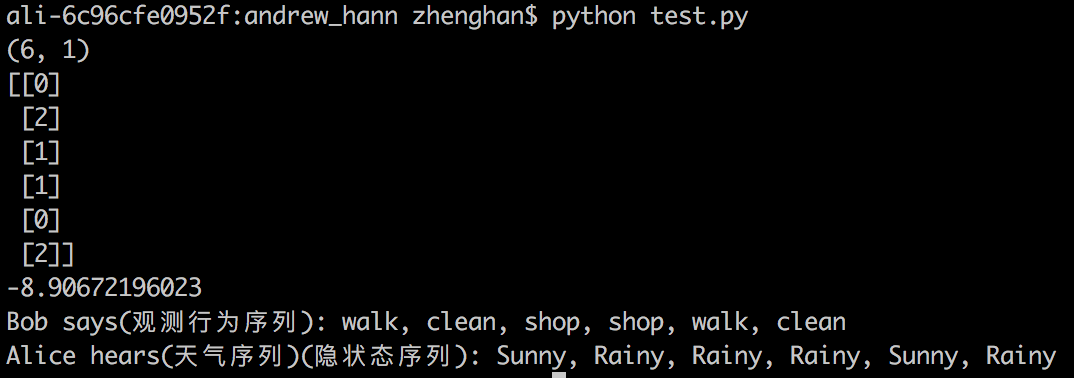http://www.cs.ubc.ca/~murphyk/Bayes/rabiner.pdf
http://www.cnblogs.com/pinard/p/7001397.html
http://blog.csdn.net/u014365862/article/details/50412978
http://blog.csdn.net/yywan1314520/article/details/50533850
http://blog.csdn.net/wowotuo/article/details/40783357

## 0x3：学习问题和概率计算问题搭配使用 - 先根据观测数据（训练样本）train出一个模型（包含模型参数），然后基于这个模型预测新的样本（观测变量）的出现概率（即异常判断）

import numpy as np
from hmmlearn.hmm import GaussianHMM

###############################################################################
# Working with multiple sequences
X1 = [[0.5], [1.0], [-1.0], [0.42], [0.24]]
X2 = [[2.4], [4.2], [0.5], [-0.24]]

# concatenate them into a single array and then compute an array of sequence lengths:
X = np.concatenate([X1, X2])
lengths = [len(X1), len(X2)]

###############################################################################
# Run Gaussian HMM
print "fitting to HMM ..."

# Make an HMM instance and execute fit
# There is 4 type of states
model = GaussianHMM(n_components=4, n_iter=100).fit(X)

# Train the model parameters and hidden state
model_pre = model.fit(X, lengths)

###############################################################################
print "Compute the observation sequence log probability under the model parameters"
print model_pre.score(X1)

print "Compute the log probability under the model and compute posteriors."
print model_pre.score_samples(X1)

-2.64250783257

## 0x4：学习问题和解码问题搭配使用 - 先根据观测数据（训练样本）train出一个模型（包含模型参数），然后基于这个模型预测在给定观测序列的情况下，最大似然的隐变量序列（及语音解码识别、解码问题）

import numpy as np
from hmmlearn.hmm import GaussianHMM

###############################################################################
# Working with multiple sequences
X1 = [[0.5], [1.0], [-1.0], [0.42], [0.24]]
X2 = [[2.4], [4.2], [0.5], [-0.24]]

# concatenate them into a single array and then compute an array of sequence lengths:
X = np.concatenate([X1, X2])
lengths = [len(X1), len(X2)]

###############################################################################
# Run Gaussian HMM
print "fitting to HMM ..."

# Make an HMM instance and execute fit
# There is 4 type of states
model = GaussianHMM(n_components=4, n_iter=100).fit(X)

# Train the model parameters and hidden state
model_pre = model.fit(X, lengths)

###############################################################################
print "Find most likely state sequence corresponding to X."
print model_pre.decode(X1)

print "pridect the mostly likly hidden state sequence according to the sample"
print model_pre.predict(X1)

print "Compute the posterior probability for each state in the model."
print model_pre.predict_proba(X1)



[[  4.28596850e-013   0.00000000e+000   1.00000000e+000   4.82112557e-178]
[  1.00000000e+000   1.82778069e-311   3.61988083e-021   0.00000000e+000]
[  1.00000000e+000   0.00000000e+000   7.31100741e-098   0.00000000e+000]
[  7.17255159e-002   0.00000000e+000   9.28274484e-001   0.00000000e+000]
[  9.97881894e-001   0.00000000e+000   2.11810616e-003   0.00000000e+000]]

[2 0 0 2 0]

http://hmmlearn.readthedocs.io/en/stable/api.html
http://hmmlearn.readthedocs.io/en/stable/tutorial.html 

## 0x5：基于HMM进行文本异常检测

### 1. 以白找黑

# -*- coding: utf-8 -*-

import urllib
import urlparse
import re
from hmmlearn import hmm
import numpy as np
from sklearn.externals import joblib

MIN_LEN = 6     # 处理参数值的最小长度
N_Hidden = 10    # 隐状态个数（从领域经验来看，隐状态数量和观测变量的状态数量基本上应该持平）
T = -30  # 最大似然概率阈值
# URL中会出现的特殊字符
SEN = [
'<',
'>',
',',
':',
'\'',
'/',
';',
'"',
'{',
'}',
'(',
')'
]

# 排除中文干扰 只处理127以内的字符
def isascii(istr):
if re.match(r'^(http)', istr):
return False
for i, c in enumerate(istr):
if ord(c) > 127 or ord(c) < 31:
return False
if c in SEN:
return True
return True

# 特征离散规范化，减少参数状态空间
def etl(istr):
vers = []
for i, c in enumerate(istr):
c = c.lower()
if ord(c) >= ord('a') and ord(c) <= ord('z'):   # ascii字母
vers.append([ord(c)])
elif ord(c) >= ord('0') and  ord(c) <= ord('9'):    # 数字
vers.append()
elif c in SEN:
vers.append([ord(c)])
else:
vers.append()
return np.array(vers)

def extractvec(filename):
X = []
LINES = [['']]
X_lens = 
with open(filename) as f:
for line in f:
query = urllib.unquote(line.strip('\n'))  # url解码
if len(line) >= MIN_LEN:
params = urlparse.parse_qsl(query, True)
for k, v in params:
if isascii(v) and len(v) >= N_Hidden:
vers = etl(v)  # 数据处理与特征提取
X = np.concatenate([X, vers])  # 每一个参数value作为一个特征向量
# # 通过np.concatenate整合成了一个1D长向量，同时需要额外传入len list来标明每个序列的长度边界
X_lens.append(len(vers))  # 长度
LINES.append(v)
return X, X_lens, LINES

def train(filename):
X, X_lens, LINES = extractvec(filename)
remodel = hmm.GaussianHMM(n_components=N_Hidden, covariance_type="full", n_iter=100)
remodel.fit(X, X_lens)
joblib.dump(remodel, "../model/xss-train.pkl")
return remodel

def predict(filename):
X, X_lens, LINES = extractvec(filename)
LINES = LINES[1:]
X = X[1:, :]
X_lens = X_lens[1:]
lastindex = 0
for i in range(len(X_lens)):
line = np.array(X[lastindex:lastindex+X_lens[i], :])
lastindex += X_lens[i]
pros = remodel.score(line)
if pros < T:
print "Find bad sample POR: %d LINE:%s" % (pros, LINES[i])

if __name__ == '__main__':
# 以白找黑，给HMM输入纯白样本，让其记住正常url参数的模型转化概率
# remodel = train("../data/web-attack/normal-10000.txt")    # train a GMM model with train sample

# 输入待检测样本，设定一个阈值(score的分值越小，说明出现的概率越小，也即说明偏离正常样本的程度)
predict("../data/xss-20.txt")
# predict the probability of the new sample according to the model(abnormal detection)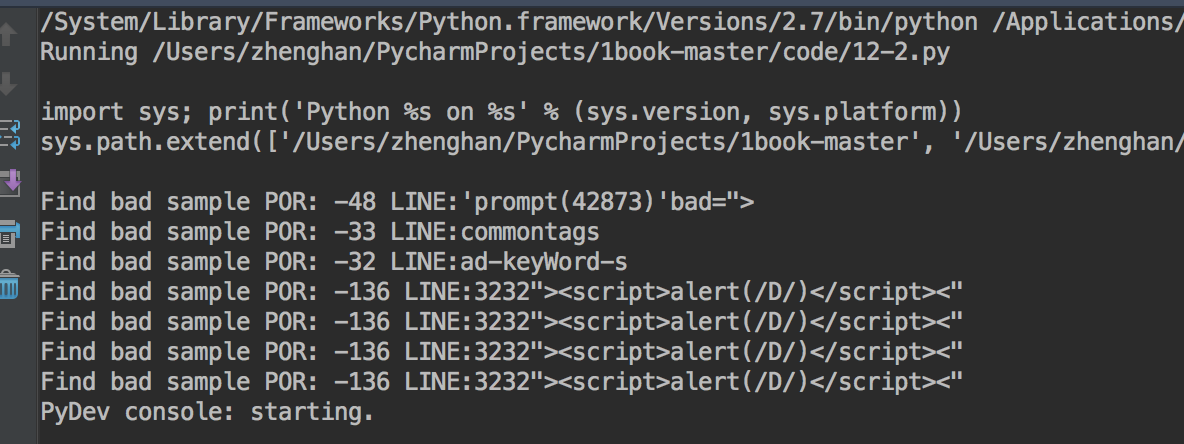### 2. 以黑找黑

# -*- coding:utf-8 -*-

import sys
import urllib
import urlparse
import re
from hmmlearn import hmm
import numpy as np
from sklearn.externals import joblib
import HTMLParser
import nltk

MIN_LEN = 10    # 处理参数值的最小长度
N = 5   # 状态个数
T = -200    # 最大似然概率阈值
SEN = ['<', '>', ',', ':', '\'', '/', ';', '"', '{', '}', '(', ')']  #其他字符2
index_wordbag = 1     # 词袋索引
wordbag = {}    # 词袋

# 数据提取与特征提取，这一步我们不采用单字符的char特征提取，而是根据领域经验对特定的phrase字符组为基础单位，进行特征化，这是一种token切分方案

# <;IFRAME SRC=http://ha.ckers.org/scriptlet.html <;
tokens_pattern = r'''(?x)
"[^"]+"
|http://\S+
|</\w+>
|<\w+>
|<\w+
|\w+=
|>
|\w+$[^<]+$ #函数 比如alert(String.fromCharCode(88,83,83))
|\w+
'''

def split_tokens(line, tokens_pattern):
tokens = nltk.regexp_tokenize(line, tokens_pattern)

X = []
X_lens = 
tokens_list = []
global wordbag
global index_wordbag

with open(filename) as f:
for line in f:
line = line.strip('\n')
line = urllib.unquote(line)   # url解码
#h = HTMLParser.HTMLParser()    # 处理html转义字符
#line = h.unescape(line)
if len(line) >= MIN_LEN:  # 忽略短url value
line, number = re.subn(r'\d+', "8", line)   # 数字常量替换成8
line, number = re.subn(r'(http|https)://[a-zA-Z0-9\.@&/#!#\?:=]+', "http://u", line)    # ulr日换成http://u
line, number = re.subn(r'\/\*.?\*\/', "", line)     # 去除注释
tokens = split_tokens(line, tokens_pattern)  # token切分
tokens_list += tokens

fredist = nltk.FreqDist(tokens_list)  # 单文件词频
keys = fredist.keys()
keys = keys[:max]     # 降维，只提取前N个高频使用的单词，其余规范化到0
for localkey in keys:  # 获取统计后的不重复词集
if localkey in wordbag.keys():  # 判断该词是否已在词袋中
continue
else:
wordbag[localkey] = index_wordbag
index_wordbag += 1
print "GET wordbag", wordbag
print "GET wordbag size(%d)" % index_wordbag

def train(filename):
X = [[-1]]
X_lens = 
global wordbag
global index_wordbag

with open(filename) as f:
for line in f:
line = line.strip('\n')
line = urllib.unquote(line)   # url解码
#h = HTMLParser.HTMLParser() # 处理html转义字符
#line = h.unescape(line)
if len(line) >= MIN_LEN:
line, number = re.subn(r'\d+', "8", line)   # 数字常量替换成8
line, number = re.subn(r'(http|https)://[a-zA-Z0-9\.@&/#!#\?:]+', "http://u", line)     # ulr日换成http://u
line, number = re.subn(r'\/\*.?\*\/', "", line)     # 干掉注释
words = split_tokens(line, tokens_pattern)
vers = []
for word in words:
# 根据词汇编码表进行index编码，对不在词汇表中的token词不予编码
if word in wordbag.keys():
vers.append([wordbag[word]])
else:
vers.append([-1])

np_vers = np.array(vers)
X = np.concatenate([X, np_vers])
X_lens.append(len(np_vers))

remodel = hmm.GaussianHMM(n_components=N, covariance_type="full", n_iter=100)
remodel.fit(X, X_lens)
joblib.dump(remodel, "../model/find_black_with_black-xss-train.pkl")

return remodel

def test(remodel, filename):
with open(filename) as f:
for line in f:
line = line.strip('\n')
line = urllib.unquote(line)     # url解码
# 处理html转义字符
#h = HTMLParser.HTMLParser()
#line = h.unescape(line)

if len(line) >= MIN_LEN:
line, number = re.subn(r'\d+', "8", line)      # 数字常量替换成8
line, number = re.subn(r'(http|https)://[a-zA-Z0-9\.@&/#!#\?:]+', "http://u", line)     # ulr日换成http://u
line, number = re.subn(r'\/\*.?\*\/', "", line)     # 干掉注释
words = split_tokens(line)
vers = []
for word in words:
# test和train保持相同的编码方式
if word in wordbag.keys():
vers.append([wordbag[word]])
else:
vers.append([-1])

np_vers = np.array(vers)
pro = remodel.score(np_vers)
if pro >= T:
print "SCORE:(%d) XSS_URL:(%s) " % (pro, line)

if __name__ == '__main__':
train_filename = "../data/xss-200000.txt"
# 基于训练样本集（纯黑，我们这里是以黑找黑），得到词频编码表

# 基于同样的训练样本集（纯黑，我们这里是以黑找黑），训练HMM模型
remodel = train(train_filename)

# 基于训练得到的HMM模型，对未知样本的最大似然概率进行预测（即预测未知样本观测序列出现的可能性，即找类似的黑样本）
test(remodel, "../data/xss-20.txt")

泛化 -> 分词 -> 词集编码 -> 词集模型处理流程

## 0x6：将HMM的对数似然概率预测值作为特征

# -*- coding:utf-8 -*-

import sys
import urllib
import urlparse
import re
from hmmlearn import hmm
import numpy as np
from sklearn.externals import joblib
import HTMLParser
import nltk
import csv
import matplotlib.pyplot as plt

MIN_LEN = 10    # 处理域名的最小长度
N = 8     # 状态个数
T = -50   # 最大似然概率阈值
FILE_MODEL = "../model/12-4.m"     # 模型文件名

domain_list = []
domain = row
if domain >= MIN_LEN:
domain_list.append(domain)
return domain_list

def domain2ver(domain):
ver = []
for i in range(0, len(domain)):
ver.append([ord(domain[i])])
return ver

def train_hmm(domain_list):
X = []
X_lens = 
for domain in domain_list:
ver = domain2ver(domain)    # 逐字符ascii向量化
np_ver = np.array(ver)
X = np.concatenate([X, np_ver])
X_lens.append(len(np_ver))

remodel = hmm.GaussianHMM(n_components=N, covariance_type="full", n_iter=100)
remodel.fit(X, X_lens)
joblib.dump(remodel, FILE_MODEL)

return remodel

domain_list = []
# xsxqeadsbgvpdke.co.uk,Domain used by Cryptolocker - Flashback DGA for 13 Apr 2017,2017-04-13,
# http://osint.bambenekconsulting.com/manual/cl.txt
with open(filename) as f:
for line in f:
domain = line.split(",")
if domain >= MIN_LEN:
domain_list.append(domain)
return domain_list

def test_dga(remodel,filename):
x = []
y = []
for domain in dga_cryptolocke_list:
domain_ver = domain2ver(domain)
np_ver = np.array(domain_ver)
pro = remodel.score(np_ver)
x.append(len(domain))
y.append(pro)
return x, y

def test_alexa(remodel,filename):
x=[]
y=[]
for domain in alexa_list:
domain_ver = domain2ver(domain)
np_ver = np.array(domain_ver)
pro = remodel.score(np_ver)
x.append(len(domain))
y.append(pro)
return x, y

if __name__ == '__main__':
# remodel = train_hmm(domain_list)    # 以白找黑: 基于alexa域名数据训练hmm模型
x_3, y_3 = test_dga(remodel, "../data/dga-post-tovar-goz-1000.txt")
x_2, y_2 = test_dga(remodel,"../data/dga-cryptolocke-1000.txt")
fig, ax = plt.subplots()
ax.set_xlabel('Domain Length')      # 横轴是域名长度
ax.set_ylabel('HMM Score')          # 纵轴是hmm对观测序列计算得到的似然概率
ax.scatter(x_3, y_3, color='b', label="dga_post-tovar-goz")
ax.scatter(x_2, y_2, color='g', label="dga_cryptolock")
ax.legend(loc='right')
plt.show()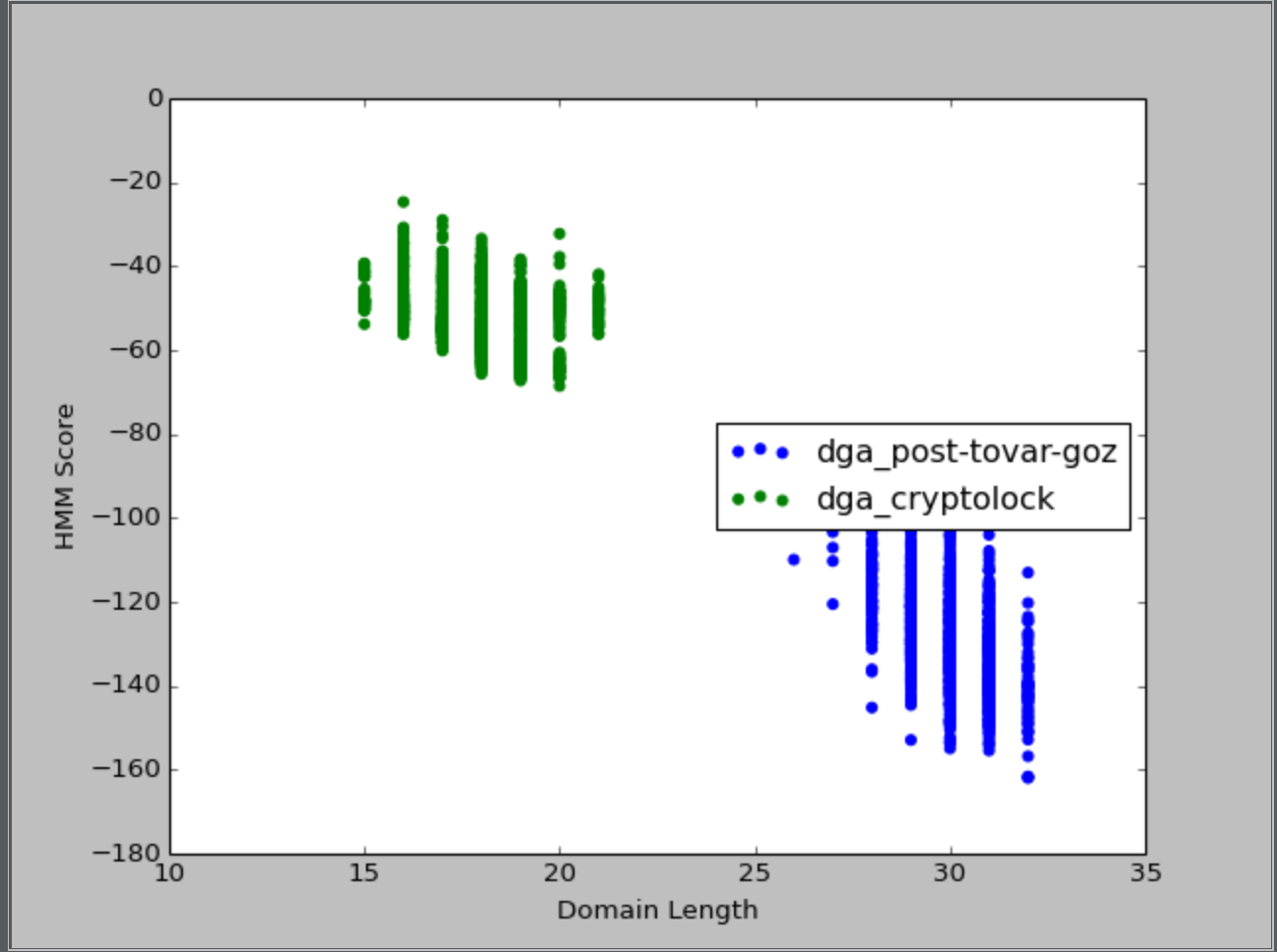posted @ 2017-10-14 22:41  郑瀚Andrew.Hann  阅读(2828)  评论(0编辑  收藏  举报